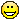0
|
3024 Views
|
3 Replies
|
7 Total Likes
View groups...
Share
GROUPS:

# Parametric force visualization

Posted 11 years ago
 Hello all.As the title says I am new to this; and have been able to stumble along on quite well so far.However, I wanted to produce a parametric representation of a few simple vectors - not field -, Any ideas other than just drawing them? Many thanks in advance for any helpKristian
3 Replies
Sort By:
Posted 11 years ago
 Hello Kristian,Here is an example that I think is useful for understanding parameterized vectors and surfaces.Here is a surface---it takes two parameters and gives you back a single vector in 3D:surface[{u_, v_}] := {Cos[u] Sin[v], Cos[v] + Sin[u], Sin[u] Sin[v]}Here is a graphical representation of that surface:surfaceGraphic = ParametricPlot3D[surface[{u, v}], {u, 0, 2 Pi}, {v, 0, 2 Pi}, PlotStyle -> Opacity[0.5], Mesh -> False]Here is a curve, it is just a simple circle; it takes one parameter and gives you a point in two dimensions.curve[t_] := {Cos[t] , Sin[t]}Let's embed the curve on the surface. We will let the curve  (i.e, vectors) play the role of u and v on the surface:curveGraphic = ParametricPlot3D[surface[curve[t]], {t, 0, 2 Pi}, PlotStyle -> Thick]Let's put those together:Show[surfaceGraphic, curveGraphic]Finally, let's see how the curve (i.e., your parameterized vector) moves along its trajectory as the parameter changes:Manipulate[ Show[surfaceGraphic, ParametricPlot3D[surface[curve[t]], {t, 0, endPoint}, PlotStyle -> { Thick, Red}], Graphics3D[{Black, Arrow[Tube[{{0, 0, 0}, surface[curve[endPoint]]}, .005]]}]], {{endPoint, Pi}, 0.0001, 2 Pi} ]Have some fun and change the surface and the curve!Craig
Posted 11 years ago
 Thanks CraigI'll have a go nowKristian
Posted 11 years ago
 This is some beautiful coding Craig. I took a liberty to add some images - I hope this is alright with you.